Pulkit Sharma — Published On July 23, 2020 and Last Modified On December 23rd, 2020

## Overview

• Get an overview of PyTorch and TensorFlow
• Learn to build a Convolutional Neural Network (CNN) model in PyTorch to solve an Image Classification problem
• Learn to build a CNN model in TensorFlow to solve an Image Classification problem

## Introduction

Image classification is one of the most important applications of computer vision. Its applications ranges from classifying objects in self driving cars to identifying blood cells in healthcare industry, from identifying defective items in manufacturing industry to build a system that can classify persons wearing masks or not. Image Classification is used in one way or the other in all these industries. How do they do it? Which framework do they use?

You must have read a lot about the differences between different deep learning frameworks including TensorFlow, PyTorch, Keras, and many more. TensorFlow and PyTorch are undoubtedly the most popular frameworks out of all in the industry. I am sure you will find endless resources to learn the similarities and differences between these deep learning frameworks.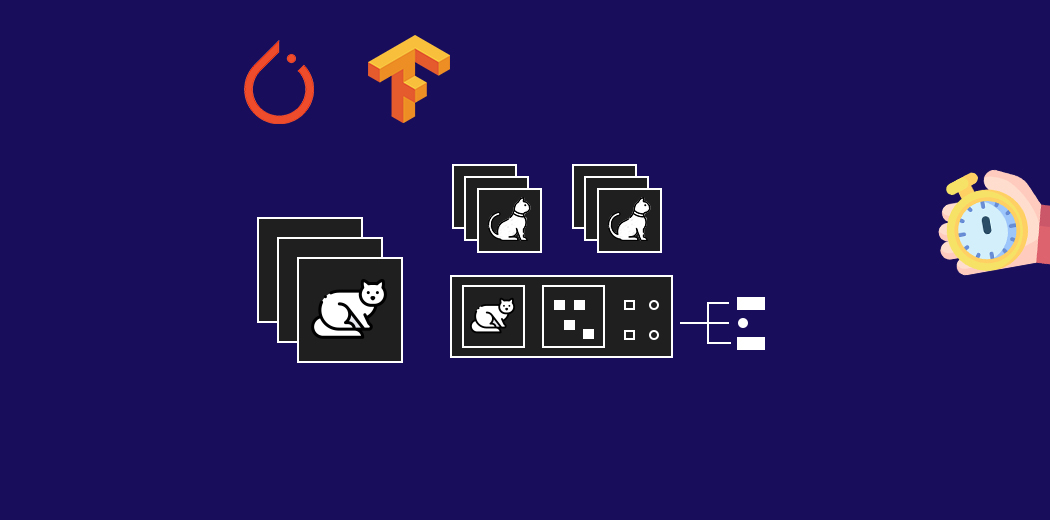In this article, we will understand how to build a basic image classification model in PyTorch and TensorFlow. We will start with a brief overview of both PyTorch and TensorFlow. And then we will take the benchmark MNIST handwritten digit classification dataset and build an image classification model using CNN (Convolutional Neural Network) in PyTorch and TensorFlow.

This will act as a starting point for you and then you can pick any of the frameworks which you feel comfortable with and start building other computer vision models too.

If you’re new to deep learning and are fascinated by the field of computer vision (who isn’t?!), do check out the ‘Certified Computer Vision Master’s Program‘.

1. Overview of PyTorch
2. Overview of TensorFlow
3. Understanding the Problem Statement: MNIST
4. Implementing a CNN in PyTorch
5. Implementing a CNN in TensorFlow

## Overview of PyTorch

PyTorch is gaining popularity in the Deep Learning community and is widely being used by deep learning practitioners, PyTorch is a Python package that provides Tensor computations. Furthermore, tensors are multidimensional arrays just like NumPy’s ndarrays which can run on GPU as well.

A distinct feature of PyTorch is that it uses dynamic computation graphs. The Autograd package of PyTorch builds computation graphs from tensors and automatically computes gradients. Instead of predefined graphs with specific functionalities.

PyTorch provides a framework for us to build computational graphs as we go, and even change them during runtime. Particularly, this is valuable for situations where we don’t know how much memory for creating a neural network. You can work on all sorts of deep learning challenges using PyTorch. The following are some of the challenges:

1. Images (Detection, Classification, etc.)
2. Text (classification, generation, etc.)
3. Reinforcement Learning

The following are some detailed resources if you wish to learn about PyTorch from scratch:

## Overview of TensorFlow

TensorFlow was developed by researchers and engineers from the Google Brain team. It is far away from the most commonly used software library in the field of deep learning (though others are catching up quickly).

One of the biggest reasons TensorFlow is so popular is its support for multiple languages to create deep learning models, such as Python, C++, and R. It has detailed documentations and walkthroughs for guidance.

There are numerous components that go into making TensorFlow. The following are the two standout ones are:

1. TensorBoard: Helps in effective data visualization using data flow graphs
2. TensorFlow: Useful for rapid deployment of new algorithms/experiments

TensorFlow is currently running version 2.0 which was officially released in September 2019. We will also be implementing CNN in 2.0 version.

I hope you now have a basic understanding of both PyTorch and TensorFlow. Now, let’s try to build a deep learning model using these two frameworks and understand their internal working. Before that let’s first understand the problem statement that we will be solving in this article.

## Understanding the Problem Statement: MNIST

Before we begin, let us understand the dataset.  In this article, we will be solving the popular MNIST problem. It is a digit recognition task wherein we have to classify the images of handwritten digits into either of the 10 classes which are  0 to 9.

In the MNIST dataset, we have images of digits that were taken from a variety of scanned documents, normalized in size, and centered. Subsequently, each image is a 28 by 28-pixel square (784 pixels total). A standard split of the dataset is used to evaluate and compare models, where 60,000 images are used to train a model and a separate set of 10,000 images are used to test it.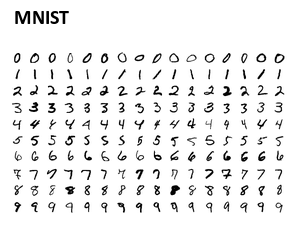Now, we have understood the dataset as well. So, let’s build our image classification model using CNN in PyTorch and TensorFlow. We will start with implementation in PyTorch. We will be implementing these models in google colab which provides free GPU to run these deep learning models.

I hope you are familiar with Convolutional Neural Networks (CNNs), if not, feel free to refer to the following article:

## Implementing a CNN in PyTorch

Let’s also check the version of PyTorch on google colab: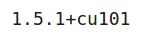So, I am using the 1.5.1 version of PyTorch. If you are using any other version, you might get a few warnings or errors, so you can update to this version of PyTorch. We will perform some transformations on the images, like normalizing the pixel values, so, let’s define those transformations as well:

Now, let’s load the training and testing set of the MNIST dataset:

Next, I have defined the train and test loader which will help us to load the training and test set in batches. I will define the batch size as 64:

Let’s look at the summary of the training set first: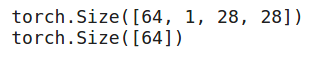So, in each batch, we have 64 images, each of size 28,28 and for each image, we have a corresponding label. Let’s visualize a training image and see how it looks: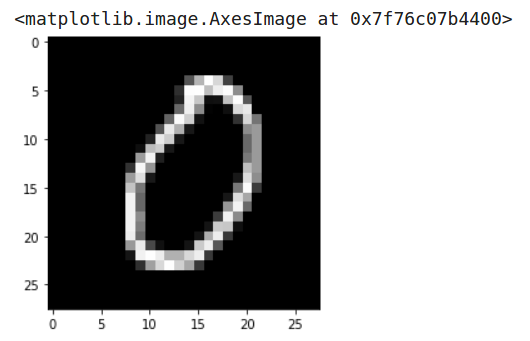It’s an image of number 0. Similarly, let’s visualize the test set image: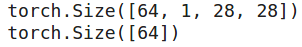In the test set also, we have batches of size 64. Let’s now define the architecture

### Defining Model Architecture

We will be using a CNN model here. So let us define and train this model:

Let’s also define the optimizer and loss function then we will look at the summary of this model: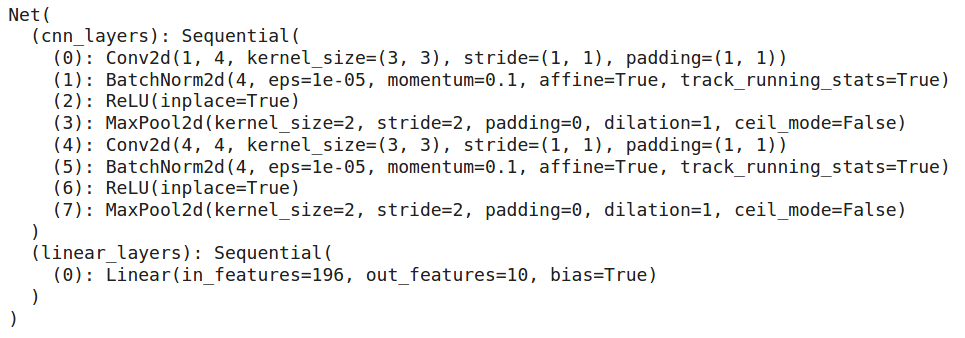So, we have 2 convolutional layers that will help to extract features from the images. Features from these convolutional layers are passed to the fully connected layer which classifies the images into their respective class. Now our model architecture is ready, let’s train this model for 10 epochs: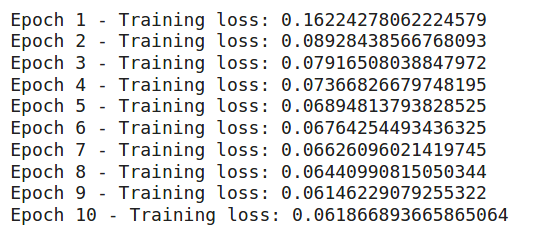You can see that the training is decreasing with an increasing number of epochs. This means that our model is learning patterns from the training set. Let’s check the performance of this model on the test set: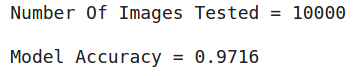So, we tested a total of 10000 images and the model is around 96% accurate in predicting the labels for test images. This is how you can build a Convolutional Neural Network in PyTorch. In the next section, we will look at how to implement the same architecture in TensorFlow.

## Implementing a CNN in TensorFlow

Now, let’s solve the same MNIST problem using a CNN in TensorFlow. As always, we will start with importing the libraries:

Let’s also check the version of TensorFlow that we are using: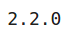So, we are using the 2.2.0 version of TensorFlow. Let’s now load the MNIST dataset using the datasets class of tensorflow.keras:

Here, we have loaded the training as well as the test set of the MNIST dataset. Also, we have normalized the pixel values for both training as well as test images. Next, let’s visualize a few images from the dataset: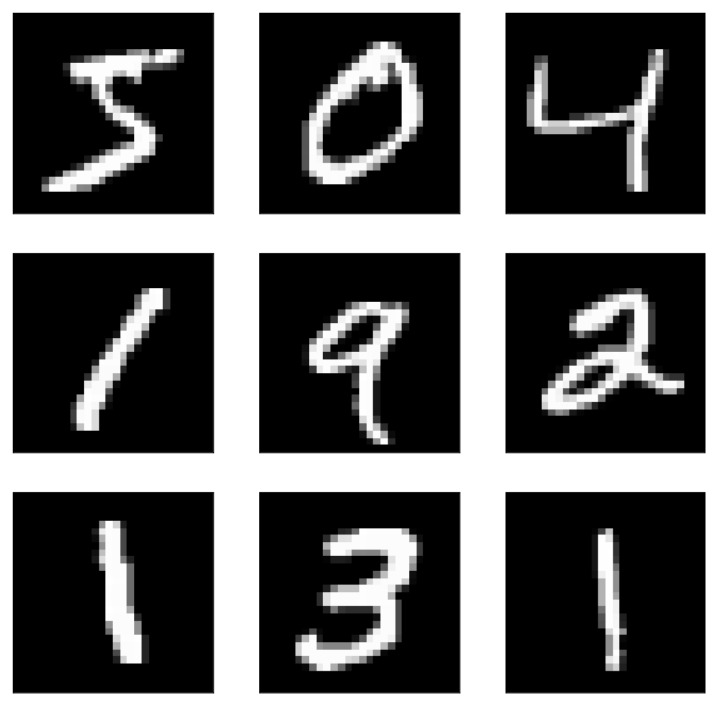Subsequently, this is how our dataset looks like. We have images of handwritten digits. Let’s also look at the shapes of the training and test set: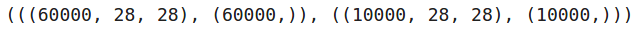So, we have 60,000 images of shape 28 by 28 in the training set and 10,000 images of the same shape in the test set. Next, we will resize the shape of images and one-hot encode the target variable:

### Defining Model Architecture

Now, we will define the architecture of our model. We will use the same architecture which we defined in PyTorch. So, our model will have 2 convolutional layers, with a combination of max-pooling layers, then we will have a flatten layer and finally a dense layer with 10 neurons since we have 10 classes.

Let’s quickly look at the summary of the model: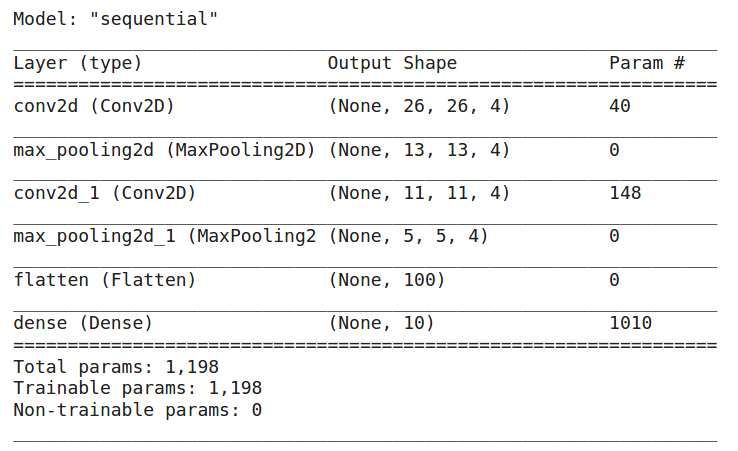To summarize, we have 2 convolutional layers, 2 max-pooling layers, a flatten layer, and a dense layer. The total number of parameters in the model is 1,198. Now that our model is ready, we will compile it:

We are using Adam optimizer, and you can change it as well. The loss function is set to be as categorical cross-entropy since we are solving a multi-class classification problem and the metric is accuracy. Now let’s train our model for 10 epochs: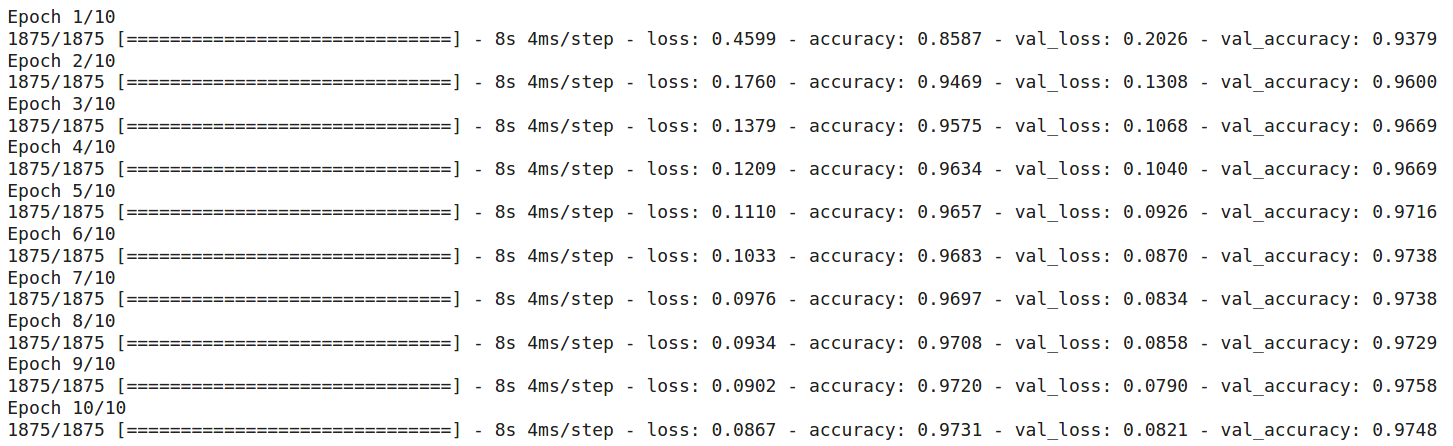To summarize, initially, the training loss was about 0.46 and after 10 epochs, the training loss reduced to 0.08. The training and validation accuracies after 10 epochs are 97.31% and 97.48% respectively.

So, that’s how we can train a CNN in TensorFlow.

## End Notes

To summarize, in this article, we first looked at a brief overview of PyTorch and TensorFlow. Then we understood the MNIST handwritten digit classification challenge and finally, build an image classification model using CNN(Convolutional Neural Network) in PyTorch and TensorFlow. Now, I hope you will be familiar with both these frameworks. As a next step, take another image classification challenge and try to solve it using both PyTorch and TensorFlow.

Here are some hackathons to practice and excel in image classification-

Do share your learning in the comments section. Also, as always, in case you have any doubts regarding this article, feel free to post them in the comments section below.